# Living By Chemistry SECOND EDITION Unit 4 TOXINS

• Slides: 13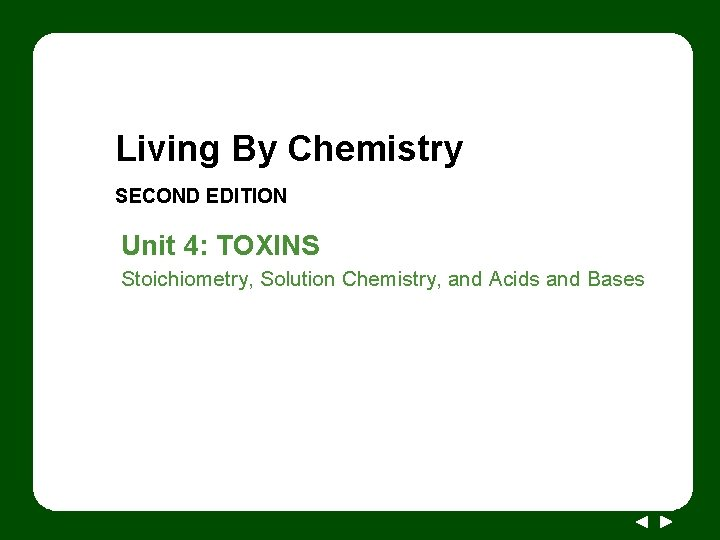Living By Chemistry SECOND EDITION Unit 4: TOXINS Stoichiometry, Solution Chemistry, and Acids and BasesLesson 89: Drip Drop Titration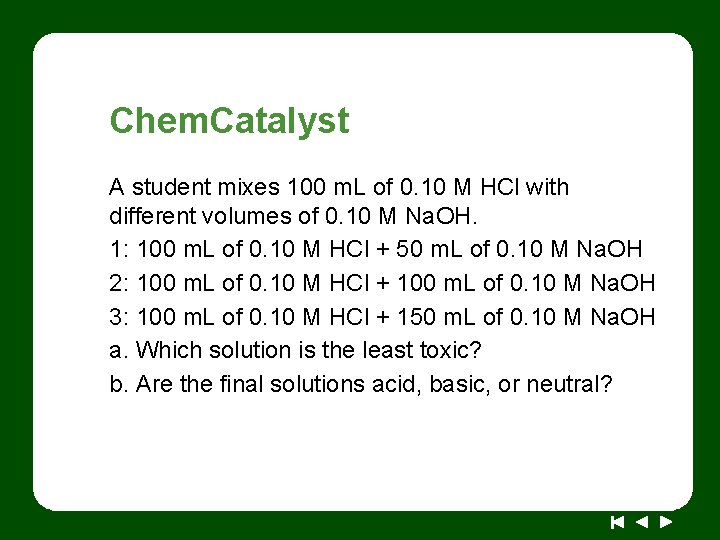Chem. Catalyst A student mixes 100 m. L of 0. 10 M HCl with different volumes of 0. 10 M Na. OH. 1: 100 m. L of 0. 10 M HCl + 50 m. L of 0. 10 M Na. OH 2: 100 m. L of 0. 10 M HCl + 100 m. L of 0. 10 M Na. OH 3: 100 m. L of 0. 10 M HCl + 150 m. L of 0. 10 M Na. OH a. Which solution is the least toxic? b. Are the final solutions acid, basic, or neutral?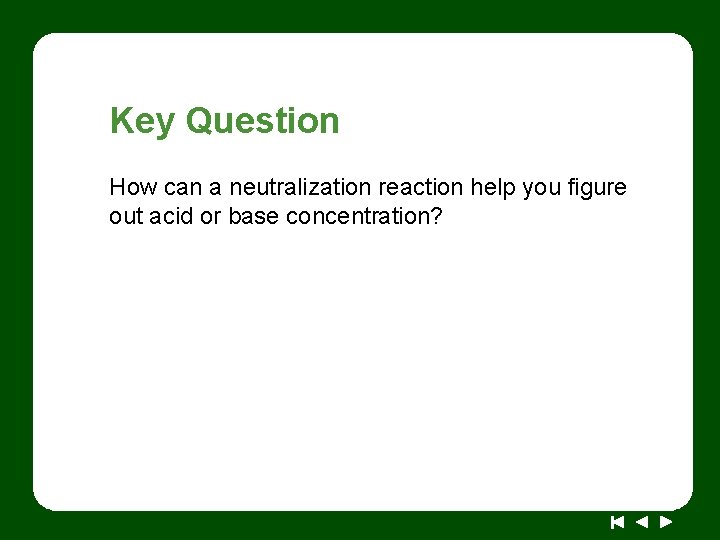Key Question How can a neutralization reaction help you figure out acid or base concentration?You will be able to: • • explain and complete a titration procedure use titration data to determine the molarity of a solution whose concentration is unknown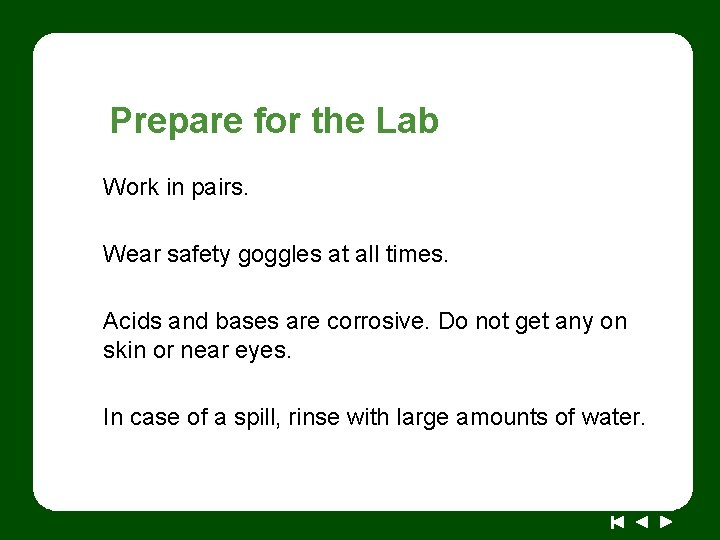Prepare for the Lab Work in pairs. Wear safety goggles at all times. Acids and bases are corrosive. Do not get any on skin or near eyes. In case of a spill, rinse with large amounts of water.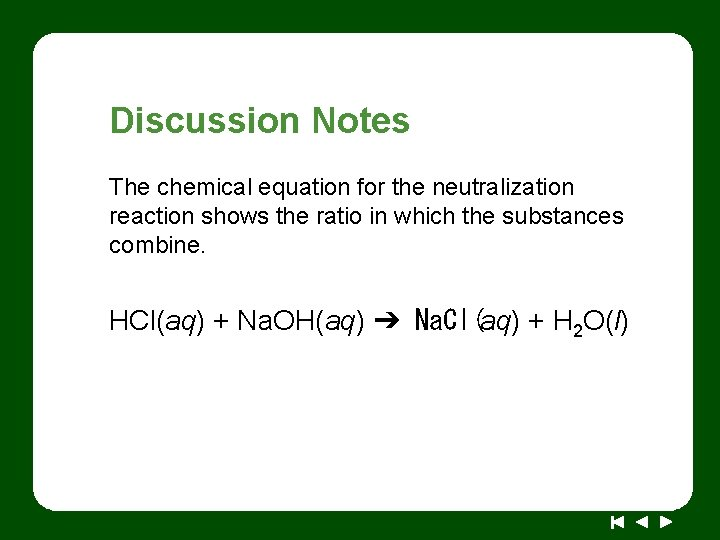Discussion Notes The chemical equation for the neutralization reaction shows the ratio in which the substances combine. HCl(aq) + Na. OH(aq) ➔ Na. Cl(aq) + H 2 O(l)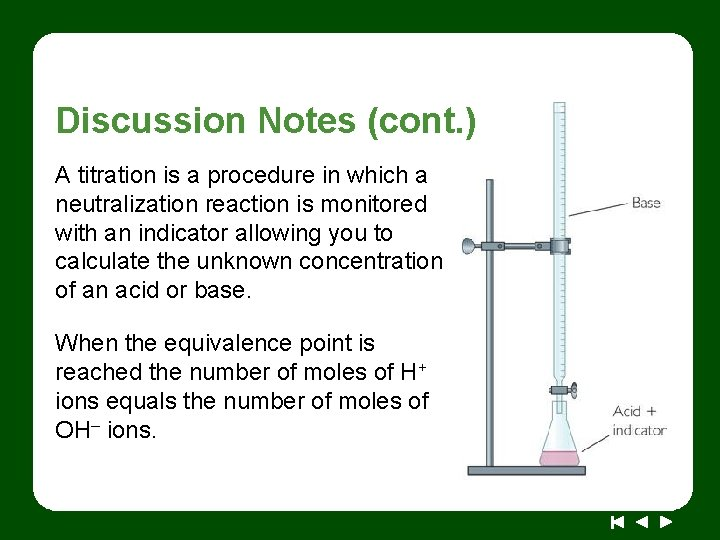Discussion Notes (cont. ) A titration is a procedure in which a neutralization reaction is monitored with an indicator allowing you to calculate the unknown concentration of an acid or base. When the equivalence point is reached the number of moles of H+ ions equals the number of moles of OH– ions.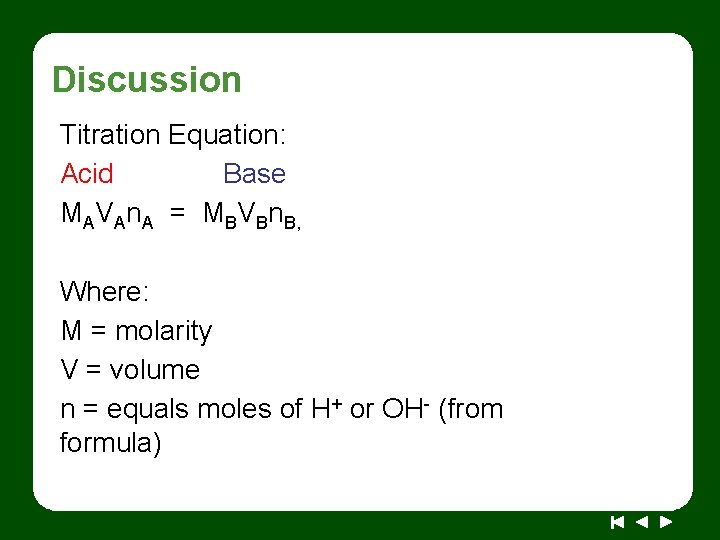Discussion Titration Equation: Acid Base MAVAn. A = MBVBn. B, Where: M = molarity V = volume n = equals moles of H+ or OH- (from formula)Titration MAVAn. A = MBVBn. B, ◆ 42. 5 m. L of 1. 3 M KOH are required to neutralize 50. 0 m. L of H 2 SO 4. Find the molarity of H 2 SO 4 + (base) OH+ (acid) H O H 3 M=? V = 50. 0 m. L n=2 M = 1. 3 M V = 42. 5 m. L n=1 MVn = MVn M(50. 0 m. L)(2) =(1. 3 M)(42. 5 m. L)(1) M = 0. 55 M H 2 SO 4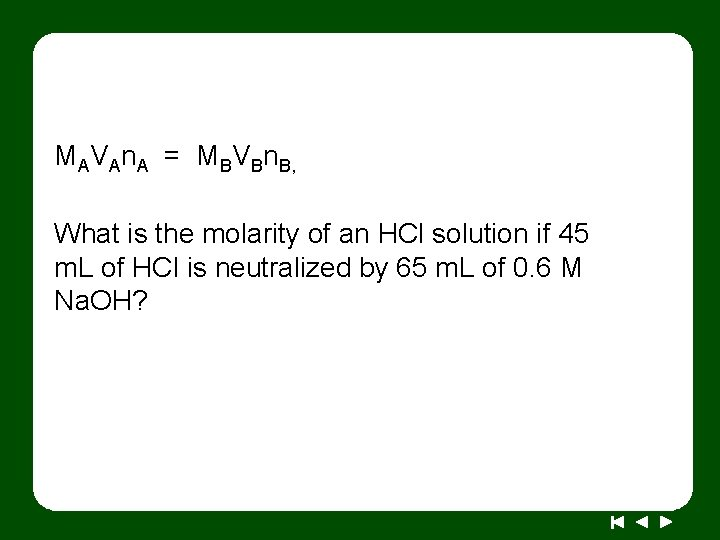MAVAn. A = MBVBn. B, What is the molarity of an HCl solution if 45 m. L of HCl is neutralized by 65 m. L of 0. 6 M Na. OH?Wrap Up How can a neutralization reaction help you figure out acid or base concentration? • A titration is a procedure that allows you to calculate the unknown concentration of an acid or a base using a neutralization reaction. • During a titration, an indicator is used to signal when the equivalence point has been reached.Check-In A beaker has 50 drops of HCl, along with a drop of phenolphthalein indicator. After 100 drops of 0. 10 M Na. OH are added, the color changes from clear to bright pink. What is the concentration of the original HCl solution?